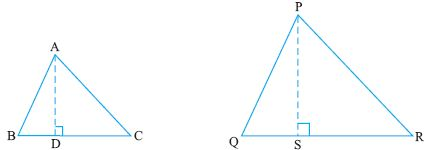Email us to get an instant 20% discount on highly effective K-12 Math & English kwizNET Programs!

#### Online Quiz (WorksheetABCD)

Questions Per Quiz = 2 4 6 8 10

### Geometry2.21 Areas of Similar Triangles

 Theorem: The ratio of the areas of similar triangles is equal to the ratio of the squares of their corresponding sides. Given: Triangle ABC and Triangle PQR are similarTo prove: (Area of triangle ABC)/(Area of triangle PQR) = AB2/PQ2 = BC2/QR2 = CA2/RP2 Construction: Draw AD perpendicular to BC and PS perpendicular to QR Proof: (Area of triangle ABC)/(Area of triangle PQR) = (1/2 x BC x AD)/(1/2 x QR x PS) or (area of ABC)/(area of PQR) = BC/QR x AD/PS ----------equation 1 Now in triangles ADB and PSQ angle B = angle Q (they are similar triangles) angle ADB = angle PSQ (90o) Hence the triangles ADB and PSQ are similar Consequently AD/PS = AB/PQ But AB/BC = BC/QR ( As ABC and PQR are similar) Hence AD/PS = BC/QR---------equation 2 Substituting 2 in 1 we get (Area of ABC)/(Area of triangle PQR) = BC/QR x BC/QR (Area of ABC)/(Area of triangle PQR) = BC2/QR Example: Prove that PM2 = QM x MRSolution: As PM is perpendicular to QR we find that triangles PMQ and PMR are similar Hence PM/MR = QM/PM (In similar triangles corresponding sides are proportional) Hence PM2 = MR x QM Directions: Solve the following problems. Also write at least 10 examples of your own.
 Q 1: In triangle ABC, BE is perpendicular to AC, AD is perpendicular to BC. Show that CDxAB = CAx DEAnswer: Q 2: ABD is a triangle in which angle DAB is 90 degrees. AC is perpendicular to DB. Prove that AB2 = BCxBDAnswer: Q 3: ABCD is a trapezium in which AB ll CD. AB = 2CD. Find the ratio of the triangles of AOB and COD.1/41/24/1 Q 4: A vertical stick 12 cm long casts a shadow 8cm long on the ground. at the same time a tower casts a shadow 40 cm on the ground. Determine the height of the tower.25 cm15 cm60 cm Q 5: In triangle ABC, XY is ll AC. XY, divides the region into two equal areas. Determine AX/AB1/21/42/1 Q 6: In triangle ABC, BE is perpendicular to AC, AD is perpendicular to BC. Show that the two triangles ADC and BEC are similar.Answer: Q 7: In triangle ABC, BE is perpendicular to AC, AD is perpendicular to BC. Show that the two triangles ABC and DEC are similarAnswer: Q 8: The areas of two similar triangles ABC and PQR are 64 cm2 and 121 cm2. If QR = 15.4 cm, find BC.29 cm25 cm26.8 cm Question 9: This question is available to subscribers only! Question 10: This question is available to subscribers only!